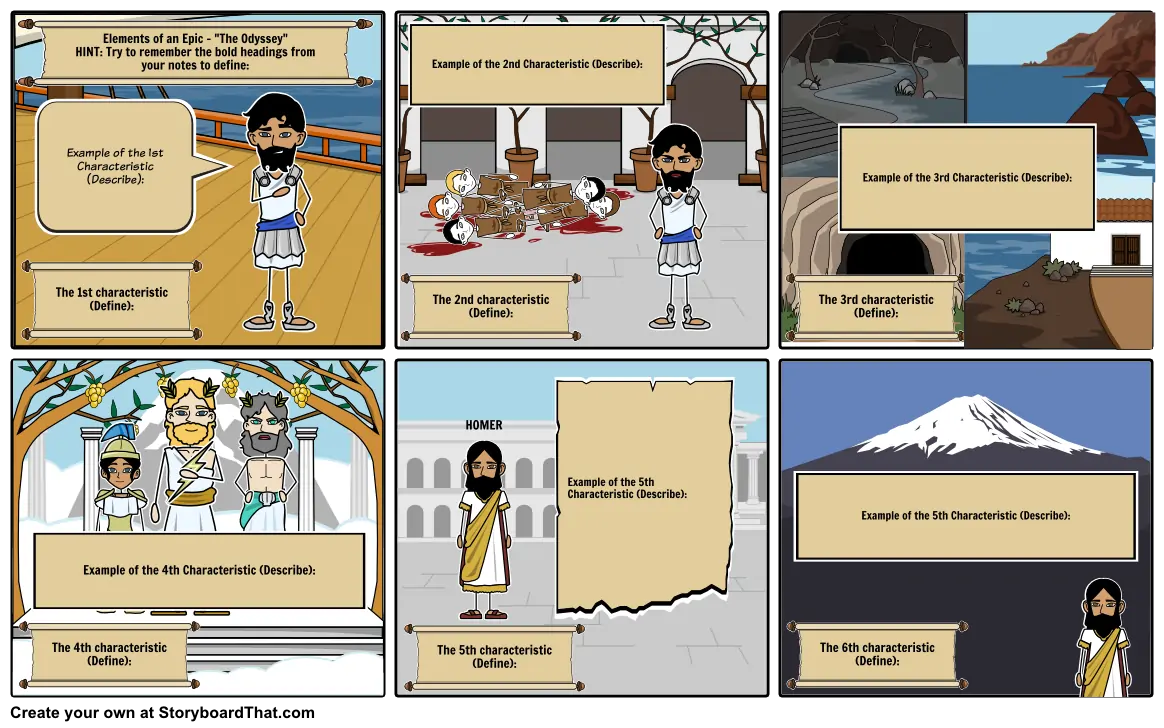# 6 Characteristics of the Epic_Odyssey#### Storyboard Text

• Elements of an Epic - "The Odyssey" HINT: Try to remember the bold headings from your notes to define:
• The 1st characteristic (Define):
• Example of the 1st Characteristic (Describe):
• The 2nd characteristic (Define):
• Example of the 2nd Characteristic (Describe):
• The 3rd characteristic (Define):
• Example of the 3rd Characteristic (Describe):
• The 4th characteristic (Define):
• Example of the 4th Characteristic (Describe):
• The 5th characteristic (Define):
• HOMER
• Example of the 5th Characteristic (Describe):
• The 6th characteristic (Define):
• Example of the 5th Characteristic (Describe):
##### Over 20 Million Storyboards Created